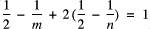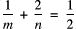Spiral Tilings

Paul Gailiunas

25 Hedley Terrace, Gosforth

Newcastle, NE3 1DP, England

Abstract

In Tilings and Patterns  Grünbaum and Shephard comment that there is extremely little literature on the subject of spiral tilings. They survey what is known, and leave some unanswered questions, in particular whether there is a tile that will produce an r-armed spiral tiling for any odd value of r ³ 5. A new method of generating tiles from regular polygons is developed here, that allows the construction of zig-zag spirals with any desired number of arms. Spiral tilings of a type already known are considered and new tilings, related to them, are described. Further new tilings are constructed by considering some special cases.

Zig-zag Spiral Tilings

Overlapping copies of any regular n-gon will produce a shape that will tile the plane (without gaps) in several different ways, one of which is a one-armed spiral, along with various two-armed spirals . If n is odd it is difficult to get much further, but if n is even then the tile that is produced can be cut into a series of rhombuses (fig.1), ensuring that each cut has the same length as a side of the polygon. Any one cut divides the original tile into two new ones, both of which will tile the plane in a variety of ways, in certain circumstances including spirals. In an n-gon it is easily verified that the angles of the rhombuses are an integer times 1/n turns (where 1 turn = 360°). In fact the rth rhombus has an angle of r/n turns, so it is possible to choose a cut that will produce a tile having one angle that is 1/m turns, if m = n/r.Figure 1: Overlapping copies of a 14-gon will produce a shape that will tile the plane.  Tiles can be produced by cutting along any of the lines indicated. Angle a = 1/14 turn, b = 2/14 = 1/7, and so on.Figure 2: A spiral begins when tiles are fitted around the convex edge of another tile (part of the n-gon). This can be done in two ways, although the new edge that is produced is the same It has a zig-zag profile.
By choosing a tile with an angle of 1/m a spiral tiling with m arms can be produced. Fitting more tiles makes the zig-zags bigger.Figure 3: A five-armed spiral from a tile based on a decagon. It has ten points.Figure 4: A five-armed spiral based on a tile from a 20-gon has twenty points. In general any zig-zag spiral based on a tile from an n-gon has n points, since the central tiles (m of them) have r edges on the convex side, each of which produces a step in the zig-zag, and m ´ r = n.
A different m-armed spiral can be produced by making the cut at the (r-1)th rhombus and placing the tile against its mirror image, rotated by a half-turn, since the apex corresponds with angle a in figure 1, having an angle of 1/n turn, and the combined angle is again the mth part of a turn.Figure 5: The same tile from a 20-gon can be used to make a
four- and a five-armed spiral, since 1/5 + 1/20 = 1/4

The alternative way of tiling the spiral arms, indicated in the fig.2 , will only work in two cases, a three-armed spiral with a tile based on a dodecagon (fig.6) and a four armed spiral with a tile from an octagon (fig.7) . The reason is seen most easily in the three-armed example.There are four solutions, m = 3, n = 12 (fig.6); m = 4, n = 8 (fig.7); m = 6, n = 6; m = 10, n = 5, but the third is the degenerate case of a tiling of triangles, and the fourth cannot be realised, since m must divide n.There are four solutions, m = 3, n = 12 (fig.6); m = 4, n = 8 (fig.7); m = 6, n = 6; m = 10, n = 5, but the third is the degenerate case of a tiling of triangles, and the fourth cannot be realised, since m must divide n.Figure 6: Two types of three-armed spiral using a tile from the dodecagon.Figure 7: Two types of four-armed spiral using a tile from the octagon.

Versatiles

In the particular cases when n is a multiple of 6 one of the rhombuses will have an angle of 1/6 turn, and it can be seen as two equilateral triangles. (Actually there are two such rhombuses, but we are only concerned with the one having the angle of 1/6 on the convex side of the n-gon.) Making the cut that divides this rhombus into triangles generates a tile (fig.8) of the type discussed by Grünbaum and Shephard, and termed a versatile in their 1979 article .Figure 8: Versatiles can fit together along the concave side of the n-gon, and along the convex side in two ways. Copies of the tile will fit into the gaps in ii) producing a profile that is the same as i). It is clear that spirals made from versatiles do not have zig-zags.

Grünbaum and Shephard showed that such tiles will always produce one-, two-, three- and six- armed spirals. It is not clear whether they realised that all except the three-armed case can be constructed using either tiling pattern i) or ii). They certainly never mention it, and they use type ii) in only one, a very attractive two-armed spiral (which is used on the dust-wrapper of their book).Figure 9: A six-armed spiral having all arms of type ii), using a tile derived from a 30-gon. The spirals can been seen as starting from six tiles fitted around a 30-gon.

The Dodecagon Versatile

In the special case when n = 12, the dodecagon, there are further possibilities. Like any versatile it will produce several two-armed, a three-armed and the two types of six-armed spiral. Rice and Schattschneider  have also described some other ways that it will tile the plane. This particular versatile also generates a four-armed and an eight-armed spiral. The four-armed spiral (fig.10) is possible because the base angles of a versatile are 1/6 turn (making the six-armed spirals possible) and (1/3-1/n) turn (making the three-armed spiral possible, by combining a tile with its mirror image). When n = 12, (1/3-1/n) = 1/4, hence a four-armed spiral is possible. The only other value of n that works to give an integer division of a full turn, n = 6, the hexagon, gives a tile that is degenerate (an equilateral triangle).Figure 10: A four-armed spiral using the dodecagon versatile.

The other possibility to consider is combining the apex (of 1/n turns) with the 1/6 angle. The solutions of 1/n + 1/6 = 1/m must have m < 6, and m = 3 is the degenerate case again. The case m = 4 has n =12, and a tiling (having eight arms) is possible (fig.11), but the tiles produced when m = 5 do not fit together in the same way, and there is no analogous tiling based on a 30-gon.Figure 11: This tiling shows particularly clearly that the spiral arms, of both
forms, are directed, since half are in one sense, half the other.

Rice and Schattschneider published this tiling (fig.11) with arms of type i), and again it is not clear if they were aware of the type ii) alternative. They also mention the existence of a twelve-armed spiral using this tile. There are many variants of this tiling (fig.12 shows one of them), since there are several ways to construct the central dodecagon. The next layer, which enlarges the dodecagon to twice its size, can be constructed in many different ways, and the spiral arms themselves can be of either type i) or ii).Figure 12: One of the many variants of a twelve-armed
spiral using the dodecagon versatile.

V-Shaped Tiles

The arms of this spiral tiling (fig.12) can be seen as originating at the mid-points of the sides of the large dodecagon, with tiles fitting around its vertices. Clearly this is only possible if the tile has no more than two concave sides, and is more or less v-shaped. The only versatile with this characteristic is derived from the dodecagon, but such tiles can be formed from any polygon. The one from the hexagon is big enough this time to produce tilings that are recognisable as spirals. Spiral tilings made with this tile (fig.13) are probably the simplest that have more than two arms, and it is surprising that they are not mentioned in the existing literature on the subject.Figure 13: The six-armed spiral follows the typical construction, being formed around a hexagon of side 2. The three armed spiral is possible because of the special properties of tiles based on the hexagon.

It is always possible to cover an n-gon of double size, using a tile derived from the n-gon, when n = 2(2r + 1), but only the decagon provides a v-shaped tile that does this. It generates a ten-armed spiral (fig.14), and of course in this case the arms are zig-zags.Figure 14: A ten-armed zig-zag spiral around a decagon of side 2.

Conclusion

Tiles that are derived from regular polygons provide the most obvious possibilities for covering the plane in ways that that appear as spirals. Zig-zag spirals can be produced with any desired number of arms by following general methods, and versatiles provide smooth spirals, although only a limited number of arms is possible. Although many special cases have been considered, it is by no means certain that there are no more left to be found.

Also the possibility of finding spiral tilings using entirely different types of tile, not based on regular polygons, has not been eliminated. Indeed some examples are discussed in , and, although further development along these lines seems unlikely, a different approach might produce more fruitful possibilities.

References

 Grünbaum B. and Shephard G.C.,Tilings and Patterns, W.H.Freeman and Company, 1987.

 Hatch G., "Tessellations with Equilateral Reflex Polygons", Mathematics Teaching, No.84, Sept.1978, p.32.

 Grünbaum B. and Shephard G.C., "Spiral Tilings and Versatiles", Mathematics Teaching, No.88, Sept.1979, pp.50-1.

 Rice M. and Schattschneider D., "The Incredible Pentagonal Versatile", Mathematics Teaching, No.93, Dec.1980, pp.52-3.

VisMath HOME